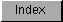```
You can use multiple Draw() statements to draw connected line figures. If
the shapes are all definable as interconnected, continuous lines, you can
use a simpler function, called PolyDraw(). PolyDraw() takes a set of line
endpoints and draws a shape using these points. You call PolyDraw() with
the statement:

PolyDraw(&rastPort, count, arraypointer);

PolyDraw() reads the array of points and draws a line from the first pair
of coordinates to the second, then a connecting line to each succeeding
pair in the array until count points have been connected. This function
uses the current drawing mode, pens, line pattern, and write mask
specified in the target RastPort; for example, this fragment draws a
rectangle, using the five defined pairs of x,y coordinates.

SHORT linearray[] =
{
3, 3,
15, 3,
15,15,
3,15,
3, 3
};

PolyDraw(&rastPort, 5, linearray);

```